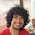### Ant Collision

Source: Anshum Agarwal (Jaadu) mailed me this problem 2-3 months back. I could solve it. :) Interesting problem.

Problem: Assume 100 ants are moving in 1 dimensional plane. All move with the same speed. Some are moving towards the positive x axis and some towards negative. If a collision occurs between two ants both ants changes the direction. If you are given direction of motion of each ant, how will you calculate the number of collisions that will occur?

1.Let's call the ants moving to right as A and those moving to left as B. For each ant belonging to A count the number of B ants on its right. Sum it over all ants in A. That's your answer.

This draws from the fact that these ants can be visualised as ghost ants meaning that when two ants collide they simply walk through each other.

2.3.the answers shud be in rot13 :(